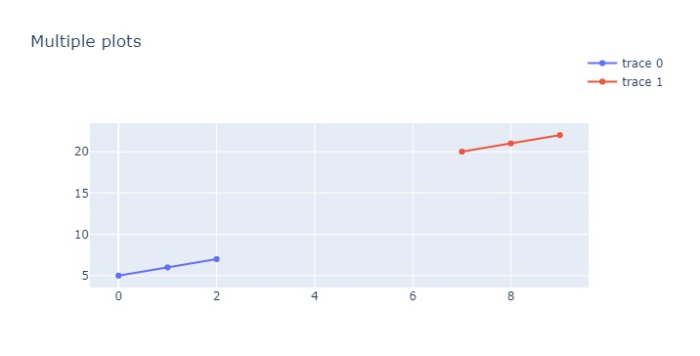# How to save multiple plots into a single HTML file in Python Plotly?

Plotly is an open-source Python library for creating charts. You can use the features available in Plotly to save multiple plots into a single HTML file. For example, plotly.subplots() method can be used to add multiple plots.

Follow the steps given to create subplots with Plotly express.

Step 1

Import plotly.graphs_objs module and alias as go

import plotly.graphs_objs as go

Step 2

Import make_subplots to create subplots.

from plotly.subplots import make_subplots

Step 3

Import plotly offline plot.

from plotly.offline import plot

Step 4

Create traces for two scatter plots and store it inside figure.

fig.add_trace(go.Scatter(x=[0, 1, 2], y=[5,6,7]), row=2, col=1)
fig.add_trace(go.Scatter(x=[7,8,9], y=[20,21,22]), row=2, col=1)

Step 5

Create the update_layout() method to set the height, width and title of the layout. It is defined below,

fig.update_layout(height=400, width=600, title_text="Multiple plots")

Step 6

Update the layout for both scatter plots in Y-axis domain. It is defined below,

fig['layout']['yaxis1'].update(domain=[0, 0.2])
fig['layout']['yaxis2'].update(domain=[0.3, 0.7])

Step 7

Generate the html file and give it a name "name.html" using offline plot method. It is defined below,

plotly.offline.plot(fig, filename='name.html')

## Example

The complete code to save multiple plots into a single HTML file in Plotly is as follows −

import plotly
from plotly.subplots import make_subplots
import plotly.graph_objects as go

fig = make_subplots(rows=3, cols=1)

fig.add_trace(go.Scatter(x=[0, 1, 2], y=[5,6,7]), row=2, col=1)

fig.update_layout(height=600, width=700,title_text="Multiple plots")
fig['layout']['yaxis1'].update(domain=[0, 0.2])
fig['layout']['yaxis2'].update(domain=[0.3, 0.7])

plotly.offline.plot(fig, filename='name.html')

## Output

It will show the following output on the browser −Also, it will save the output in your project directory as "name.html".